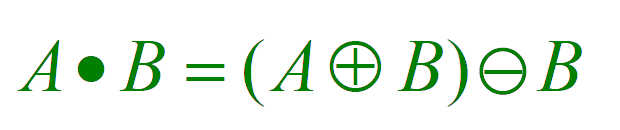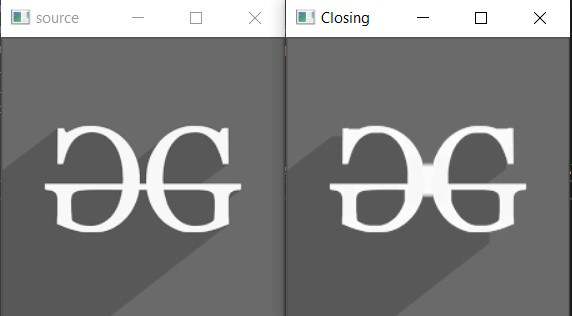Open in App
Not now

# Closing | Morphological Transformations in OpenCV in C++

• Last Updated : 10 Feb, 2021

• It helps to reduce the internal noise present inside an image.
• In this article, another operator is elaborated called closing which is just the reverse of Opening and applies dilation followed by erosion.
• Just like the Opening operator it also uses a structuring element but it is used for removing small holes instead of pertusions.

Syntax:

morphologyEx (src, dst, op, kernel, anchor, iterations, borderType, borderValue)

Parameters:

• src: It is the input image.
• dst: It is the output image.
• op: Type of morphological operation.
• kernel: Structuring element used for Closing.
• anchor: Anchor position inside the structuring element. The default value is [-1, -1} signifying position as the center of the structuring element.
• iterations: Number of times Closing is applied.
• borderType: Type of border ( BORDER_CONSTANT, BORDER_REPLICATE, etc.)
• borderValue: Border value
• Return: Output Image (Mat Object)

The closing operation is given by the expression:• The expression represents the fact that A is a sub-image of A.B.
• This operator is used to remove small holes from the image.
• It also helps in smoothening the contours and fusion of narrow breaks and long thin gulfs.

Below is the program demonstrating the Closing Morphological Operator:

## C++

 `// C++ program for demonstrating the``// closing morphological operator`` ` `// For drawing shapes``#include ``#include ``using` `namespace` `cv;``using` `namespace` `std;`` ` `// Function to demonstrate the``// closing morphological operator``void` `closingMorphological()``{``    ``// Reading the Image``    ``Mat image = imread(``        ``"C:/Users/harsh/Downloads/geeks.png"``,``        ``IMREAD_GRAYSCALE);`` ` `    ``// Check if the image is``    ``// created successfully``    ``if` `(!image.data) {``        ``cout << ``"Could not open or "``             ``<< ``"find the image\n"``;``        ``return` `0;``    ``}`` ` `    ``int` `morph_size = 2;`` ` `    ``// Create structuring element``    ``Mat element = getStructuringElement(``        ``MORPH_RECT,``        ``Size(2 * morph_size + 1,``             ``2 * morph_size + 1),``        ``Point(morph_size, morph_size));``    ``Mat output;`` ` `    ``// Closing``    ``morphologyEx(image, output,``                 ``MORPH_CLOSE, element,``                 ``Point(-1, -1), 2);`` ` `    ``// Displays the source and``    ``// the closing image formed``    ``imshow(``"source"``, image);``    ``imshow(``"Closing"``, output);``    ``waitKey();``}`` ` `// Driver Code``int` `main(``int` `argc, ``char``** argv)``{``    ``// Function Call``    ``closingMorphological();`` ` `    ``return` `0;``}`

Output:My Personal Notes arrow_drop_up